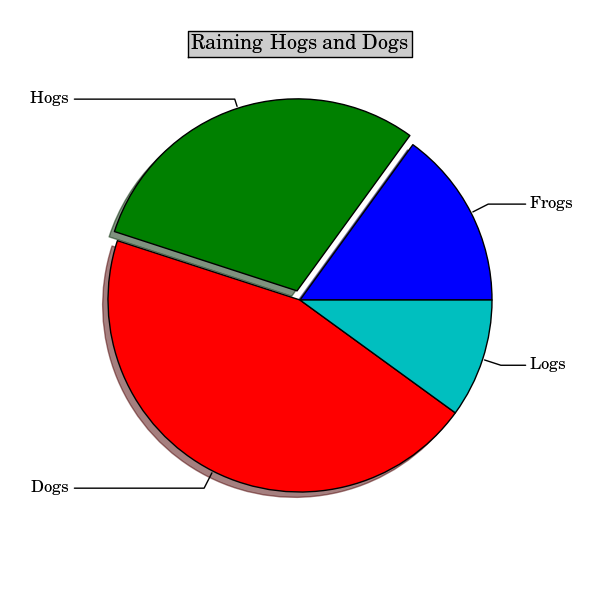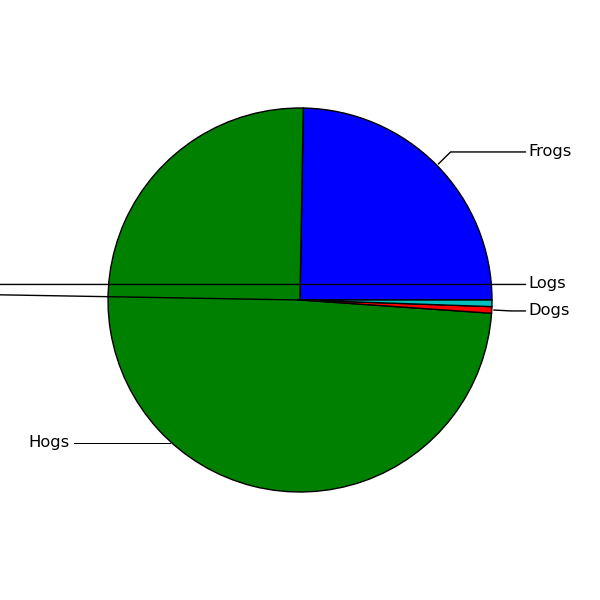# Overlapping labels in pie charts

Hello,

I am having some issues generating pie charts, when some of the slices become very small, their labels
will draw on top of each other, making it impossible to distinguish between them. And I am trying to avoid using a legend.

Does anyone know if there is a way to properly position labels of pie charts to avoid overlapping.
(By for example distributing them vertically with lines pointing to their respective slices)

Similar to what is done here:

···

Regards
Rune V. Sjoen

This should be doable using the annotation. Here is a simple cook-up I
just did. it uses a naive algorithm to place the labels, but I guess
it gives you an idea how things work.
a screenshot is attached.

Regards,

-JJ

from pylab import *

# make a square figure and axes
figure(1, figsize=(6,6))
ax = axes([0.1, 0.1, 0.8, 0.8])

labels = 'Frogs', 'Hogs', 'Dogs', 'Logs'
fracs = [15,30,45, 10]

explode=(0, 0.05, 0, 0)
title('Raining Hogs and Dogs', bbox={'facecolor':'0.8', 'pad':5})

for p1, l1 in zip(p, labels):
r = p1.r
dr = r*0.1
t1, t2 = p1.theta1, p1.theta2
theta = (t1+t2)/2.

xc, yc = r/2.*cos(theta/180.*pi), r/2.*sin(theta/180.*pi)
x1, y1 = (r+dr)*cos(theta/180.*pi), (r+dr)*sin(theta/180.*pi)
if x1 > 0 :
x1 = r+2*dr
ha, va = "left", "center"
tt = -180
cstyle="angle,angleA=0,angleB=%f"%(theta,)
else:
x1 = -(r+2*dr)
ha, va = "right", "center"
tt = 0
cstyle="angle,angleA=0,angleB=%f"%(theta,)

annotate(l1,
(xc, yc), xycoords="data",
xytext=(x1, y1), textcoords="data", ha=ha, va=va,
arrowprops=dict(arrowstyle="-",
connectionstyle=cstyle,
patchB=p1))

show()Hello again, and thank you very much for the answer, suddenly it all got much clearer to me. The only ‘issue’ I am having is (from screenshot) what happens to the line pointing to Logs when I try to offset it a little bit on the Y axis. It looks like either the angleA or angleB is wrong, but I don’t see and reason why it would be as the X coordinates does not change.

Another thing I do not quite understand is what that patchB does.

``````    figure(1, figsize=(6,6))
ax = axes([0.1, 0.1, 0.8, 0.8])

labels = 'Frogs', 'Hogs', 'Dogs', 'Logs'

fracs = [45, 135 ,1, 1]

p = pie(fracs)

foo = None
for p1, l1 in zip(p, labels):

r = p1.r
dr = r*0.1
t1, t2 = p1.theta1, p1.theta2

theta = (t1+t2)/2.

xc = cos(theta/180.*pi)*r
yc = sin(theta/180.*pi)*r
x1 = cos(theta/180.*pi)*(r+dr)
y1 = sin(theta/180.*pi)*(r+dr)

if x1 > 0 :

x1 = r+2*dr
ha, va = "left", "center"
cstyle="angle,angleA=180,angleB=%f"%(-theta,)
print >> sys.stderr, ha, ",A,", va

else:
x1 = -(r+2*dr)
ha, va = "right", "center"
cstyle="angle,angleA=0,angleB=%f"%(theta,)
print >> sys.stderr, ha, ",B,", va

if foo:
if theta - foo < 10:
print >>sys.stderr, "Overlapping, offsetting a little bit"
y1 = y1 + 0.1
foo = theta

annotate(l1,
(xc, yc), xycoords="data",
xytext=(x1, y1), textcoords="data", ha=ha, va=va,
arrowprops=dict(arrowstyle="-",

connectionstyle=cstyle,
patchB=p1))
``````
• Rune

2010/3/23 Jae-Joon Lee <lee.j.joon@…287…>···

This should be doable using the annotation. Here is a simple cook-up I

just did. it uses a naive algorithm to place the labels, but I guess

it gives you an idea how things work.

a screenshot is attached.

Regards,

-JJ

from pylab import *

# make a square figure and axes

figure(1, figsize=(6,6))

ax = axes([0.1, 0.1, 0.8, 0.8])

labels = ‘Frogs’, ‘Hogs’, ‘Dogs’, ‘Logs’

fracs = [15,30,45, 10]

explode=(0, 0.05, 0, 0)

title(‘Raining Hogs and Dogs’, bbox={‘facecolor’:‘0.8’, ‘pad’:5})

for p1, l1 in zip(p, labels):

``````r = p1.r

dr = r*0.1

t1, t2 = p1.theta1, p1.theta2

theta = (t1+t2)/2.

xc, yc = r/2.*cos(theta/180.*pi), r/2.*sin(theta/180.*pi)

x1, y1 = (r+dr)*cos(theta/180.*pi), (r+dr)*sin(theta/180.*pi)

if x1 > 0 :

x1 = r+2*dr

ha, va = "left", "center"

tt = -180

cstyle="angle,angleA=0,angleB=%f"%(theta,)

else:

x1 = -(r+2*dr)

ha, va = "right", "center"

tt = 0

cstyle="angle,angleA=0,angleB=%f"%(theta,)

annotate(l1,

(xc, yc), xycoords="data",

xytext=(x1, y1), textcoords="data", ha=ha, va=va,

arrowprops=dict(arrowstyle="-",

connectionstyle=cstyle,

patchB=p1))
``````

show()

You should not use "angle" style if you change the x,y position (this
is due to the algorithm of how the line connecting two points are
create).

if foo:
if theta - foo < 10:
print >>sys.stderr, "Overlapping, offsetting a little bit"
y1 = y1 + 0.1
if x1 > 0 :
cstyle="arc,angleA=180,armA=30,armB=10,angleB=%f"%(-theta,)
else:
cstyle="arc,angleA=0,armA=30,armB=10,angleB=%f"%(theta,)

There is not much documentation of how each algorithm works (it is
beyond my english skill). They are loosely based on the latex pstrick
package and the screenshot in the following link may be useful to get
some idea though.

http://matplotlib.sourceforge.net/users/annotations_guide.html#annotating-with-arrow

Regards,

-JJ

2010/3/24 Rune V. Sjøen <rvsjoen@...287...>:

···

Hello again, and thank you very much for the answer, suddenly it all got
much clearer to me. The only 'issue' I am having is (from screenshot) what
happens to the line pointing to Logs when I try to offset it a little bit on
the Y axis. It looks like either the angleA or angleB is wrong, but I don't
see and reason why it would be as the X coordinates does not change.

Another thing I do not quite understand is what that patchB does.

``````    figure\(1, figsize=\(6,6\)\)
ax = axes\(\[0\.1, 0\.1, 0\.8, 0\.8\]\)

labels = &#39;Frogs&#39;, &#39;Hogs&#39;, &#39;Dogs&#39;, &#39;Logs&#39;
fracs = \[45, 135 ,1, 1\]

p = pie\(fracs\)

foo = None
for p1, l1 in zip\(p\[0\], labels\):

r = p1\.r
dr = r\*0\.1
t1, t2 = p1\.theta1, p1\.theta2
theta = \(t1\+t2\)/2\.

xc = cos\(theta/180\.\*pi\)\*r
yc = sin\(theta/180\.\*pi\)\*r
x1 = cos\(theta/180\.\*pi\)\*\(r\+dr\)
y1 = sin\(theta/180\.\*pi\)\*\(r\+dr\)

if x1 &gt; 0 :
x1 = r\+2\*dr
ha, va = &quot;left&quot;, &quot;center&quot;
cstyle=&quot;angle,angleA=180,angleB=%f&quot;%\(\-theta,\)
print &gt;&gt; sys\.stderr, ha, &quot;,A,&quot;, va
else:
x1 = \-\(r\+2\*dr\)
ha, va = &quot;right&quot;, &quot;center&quot;
cstyle=&quot;angle,angleA=0,angleB=%f&quot;%\(theta,\)
print &gt;&gt; sys\.stderr, ha, &quot;,B,&quot;, va

if foo:
if theta \- foo &lt; 10:
print &gt;&gt;sys\.stderr, &quot;Overlapping, offsetting a little
``````

bit"
y1 = y1 + 0.1
foo = theta

``````       annotate\(l1,
\(xc, yc\), xycoords=&quot;data&quot;,
xytext=\(x1, y1\), textcoords=&quot;data&quot;, ha=ha, va=va,
arrowprops=dict\(arrowstyle=&quot;\-&quot;,
connectionstyle=cstyle,
patchB=p1\)\)
``````

- Rune

2010/3/23 Jae-Joon Lee <lee.j.joon@...287...>

This should be doable using the annotation. Here is a simple cook-up I
just did. it uses a naive algorithm to place the labels, but I guess
it gives you an idea how things work.
a screenshot is attached.

Regards,

-JJ

from pylab import *

# make a square figure and axes
figure(1, figsize=(6,6))
ax = axes([0.1, 0.1, 0.8, 0.8])

labels = 'Frogs', 'Hogs', 'Dogs', 'Logs'
fracs = [15,30,45, 10]

explode=(0, 0.05, 0, 0)
title('Raining Hogs and Dogs', bbox={'facecolor':'0.8', 'pad':5})

for p1, l1 in zip(p, labels):
r = p1.r
dr = r*0.1
t1, t2 = p1.theta1, p1.theta2
theta = (t1+t2)/2.

xc, yc = r/2.*cos(theta/180.*pi), r/2.*sin(theta/180.*pi)
x1, y1 = (r+dr)*cos(theta/180.*pi), (r+dr)*sin(theta/180.*pi)
if x1 > 0 :
x1 = r+2*dr
ha, va = "left", "center"
tt = -180
cstyle="angle,angleA=0,angleB=%f"%(theta,)
else:
x1 = -(r+2*dr)
ha, va = "right", "center"
tt = 0
cstyle="angle,angleA=0,angleB=%f"%(theta,)

annotate(l1,
(xc, yc), xycoords="data",
xytext=(x1, y1), textcoords="data", ha=ha, va=va,
arrowprops=dict(arrowstyle="-",
connectionstyle=cstyle,
patchB=p1))

show()

Hello again, and thanks.

I did not have a chance to look at this until now but using arc instead of
angle worked out great.

2010/3/24 Jae-Joon Lee <lee.j.joon@…287…>

···

You should not use “angle” style if you change the x,y position (this

is due to the algorithm of how the line connecting two points are

create).

if foo:

``````   if theta - foo < 10:

print >>sys.stderr, "Overlapping, offsetting a little bit"

y1 = y1 + 0.1
``````

if x1 > 0 :

``````           cstyle="arc,angleA=180,armA=30,armB=10,angleB=%f"%(-theta,)

else:

cstyle="arc,angleA=0,armA=30,armB=10,angleB=%f"%(theta,)
``````

There is not much documentation of how each algorithm works (it is

beyond my english skill). They are loosely based on the latex pstrick

package and the screenshot in the following link may be useful to get

some idea though.

http://matplotlib.sourceforge.net/users/annotations_guide.html#annotating-with-arrow

Regards,

-JJ

2010/3/24 Rune V. Sjøen <rvsjoen@…287…>:

Hello again, and thank you very much for the answer, suddenly it all got

much clearer to me. The only ‘issue’ I am having is (from screenshot) what

happens to the line pointing to Logs when I try to offset it a little bit on

the Y axis. It looks like either the angleA or angleB is wrong, but I don’t

see and reason why it would be as the X coordinates does not change.

Another thing I do not quite understand is what that patchB does.

``````    figure(1, figsize=(6,6))
``````
``````    ax = axes([0.1, 0.1, 0.8, 0.8])
``````
``````    labels = 'Frogs', 'Hogs', 'Dogs', 'Logs'
``````
``````    fracs = [45, 135 ,1, 1]
``````
``````    p = pie(fracs)
``````
``````    foo = None
``````
``````    for p1, l1 in zip(p, labels):
``````
``````       r = p1.r
``````
``````       dr = r*0.1
``````
``````       t1, t2 = p1.theta1, p1.theta2
``````
``````       theta = (t1+t2)/2.
``````
``````       xc = cos(theta/180.*pi)*r
``````
``````       yc = sin(theta/180.*pi)*r
``````
``````       x1 = cos(theta/180.*pi)*(r+dr)
``````
``````       y1 = sin(theta/180.*pi)*(r+dr)
``````
``````       if x1 > 0 :
``````
``````           x1 = r+2*dr
``````
``````           ha, va = "left", "center"
``````
``````           cstyle="angle,angleA=180,angleB=%f"%(-theta,)
``````
``````           print >> sys.stderr, ha, ",A,", va
``````
``````       else:
``````
``````           x1 = -(r+2*dr)
``````
``````           ha, va = "right", "center"
``````
``````           cstyle="angle,angleA=0,angleB=%f"%(theta,)
``````
``````           print >> sys.stderr, ha, ",B,", va
``````
``````       if foo:
``````
``````           if theta - foo < 10:
``````
``````               print >>sys.stderr, "Overlapping, offsetting a little
``````

bit"

``````               y1 = y1 + 0.1
``````
``````       foo = theta
``````
``````       annotate(l1,
``````
``````                (xc, yc), xycoords="data",
``````
``````                xytext=(x1, y1), textcoords="data", ha=ha, va=va,
``````
``````                arrowprops=dict(arrowstyle="-",
``````
``````                                connectionstyle=cstyle,
``````
``````                                patchB=p1))
``````
• Rune

2010/3/23 Jae-Joon Lee <lee.j.joon@…287…>

This should be doable using the annotation. Here is a simple cook-up I

just did. it uses a naive algorithm to place the labels, but I guess

it gives you an idea how things work.

a screenshot is attached.

Regards,

-JJ

from pylab import *

# make a square figure and axes

figure(1, figsize=(6,6))

ax = axes([0.1, 0.1, 0.8, 0.8])

labels = ‘Frogs’, ‘Hogs’, ‘Dogs’, ‘Logs’

fracs = [15,30,45, 10]

explode=(0, 0.05, 0, 0)

title(‘Raining Hogs and Dogs’, bbox={‘facecolor’:‘0.8’, ‘pad’:5})

for p1, l1 in zip(p, labels):

r = p1.r

dr = r*0.1

t1, t2 = p1.theta1, p1.theta2

theta = (t1+t2)/2.

xc, yc = r/2.*cos(theta/180.*pi), r/2.*sin(theta/180.*pi)

x1, y1 = (r+dr)*cos(theta/180.*pi), (r+dr)*sin(theta/180.*pi)

if x1 > 0 :

``````   x1 = r+2*dr
``````
``````   ha, va = "left", "center"
``````
``````   tt = -180
``````
``````   cstyle="angle,angleA=0,angleB=%f"%(theta,)
``````

else:

``````   x1 = -(r+2*dr)
``````
``````   ha, va = "right", "center"
``````
``````   tt = 0
``````
``````   cstyle="angle,angleA=0,angleB=%f"%(theta,)
``````

annotate(l1,

``````        (xc, yc), xycoords="data",
``````
``````        xytext=(x1, y1), textcoords="data", ha=ha, va=va,
``````
``````        arrowprops=dict(arrowstyle="-",
``````
``````                        connectionstyle=cstyle,
``````
``````                        patchB=p1))
``````

show()